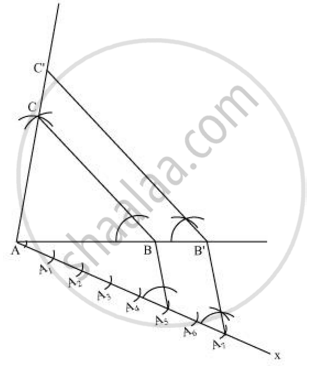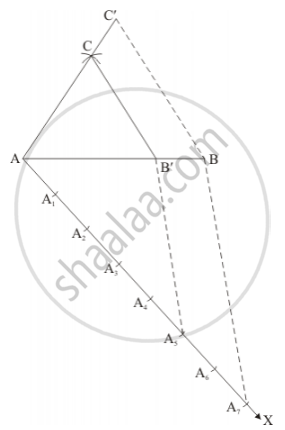# Construct a triangle with sides 5 cm, 6 cm and 7 cm and then another triangle whose sides are 7/5 of the corresponding sides of the first triangle. Give the justification of the construction. - Mathematics

Construct a triangle with sides 5 cm, 6 cm and 7 cm and then another triangle whose sides are 7/5 of the corresponding sides of the first triangle. Give the justification of the construction.

#### Solution 1

Step 1

Draw a line segment AB of 5 cm. Taking A and B as centre, draw arcs of 6 cm and 7 cm radius respectively. Let these arcs intersect each other at point C. ΔABC is the required triangle having length of sides as 5 cm, 6 cm, and 7 cm respectively.

Step 2

Draw a ray AX making acute angle with line AB on the opposite side of vertex C.

Step 3

Locate 7 points, A1, A2, A3, A4 A5, A6, A7 (as 7 is greater between 5and 7), on line AX such that AA1 = A1A2 = A2A3 = A3A4 = A4A5 = A5A6 = A6A7.

Step 4

Join BA5 and draw a line through A7 parallel to BA5 to intersect extended line segment AB at point B'.

Step 5

Draw a line through B' parallel to BC intersecting the extended line segment AC at C'. ΔAB'C' is the required triangle.Justification

The construction can be justified by proving that

AB' = 7/5AB, B'C' = 7/5(BC), AC'=7/5 (AC)

In ΔABC and ΔAB'C',

∠ABC = ∠AB'C' (Corresponding angles)

∠BAC = ∠B'AC' (Common)

∴ ΔABC ∼ ΔAB'C' (AA similarity criterion)

=> (AB)/(AB')=(BC)/(B'C')=(AC)/(AC') ....(1)

In ΔAA5B and ΔAA7B',

∠A5AB = ∠A7AB' (Common)

∠AA5B = ∠AA7B' (Corresponding angles)

∴ ΔAA5B ∼ ΔAA7B' (AA similarity criterion)

=> (AB)/(AB') = (

=>(AB)/(AB')=5/7 ....(2)

On comparing equations (1) and (2), we obtain

(AB)/(AB')=(BC)/(B'C') = (AC)/(AC') = 5/7

=>AB' = 7/5 AB, B'C' =  7/5 BC, AC' = 7/5 AC

This justifies the construction.

#### Solution 2

Given that

Construct a triangle of sides AB = 5cm, BC = 6cm and AC = 7cm and then a triangle similar to it whose sides are 7/5th of the corresponding sides of ΔABC .

We follow the following steps to construct the givenStep of construction

Step: I- First of all we draw a line segment AB = 5cm.

Step: II- With as centre and radius AC = 7cm, draw an arc.

Step: III- With as centre and radius = BC = 6cm, draw an arc, intersecting the arc drawn in step II at C.

Step: IV- Joins AC and BC to obtain ΔABC.

Step: V- Below AB, makes an acute angle ∠BAX = 60°.

Step: VI- Along AX, mark off seven points A1, A2, A3, A4, A5, A6 and A7 such that AA1 = A1A2 = A2A3 = A3A4 = A4A5 = A5A6 = A6A7

Step: VII-Join A5B.

Step: VIII- Since we have to construct a triangle each of whose sides is 7/5th of the corresponding sides of ΔABC.

So, we draw a line A3B' on AX from point A7 which is A7B' || A5B and meeting AB at B'.

Step: IX- From B' point draw B'C' || BC, and meeting ACat C'

Thus, ΔAB'C' is the required triangle, each of whose sides is 7/5th of the corresponding sides of ΔABC.

Concept: Division of a Line Segment
Is there an error in this question or solution?

#### APPEARS IN

NCERT Class 10 Maths
Chapter 11 Constructions
Exercise 11.1 | Q 3 | Page 220
RD Sharma Class 10 Maths
Chapter 9 Constructions
Exercise 9.2 | Q 5 | Page 9